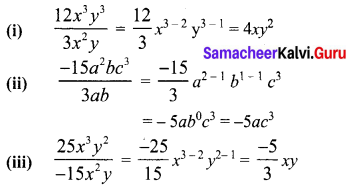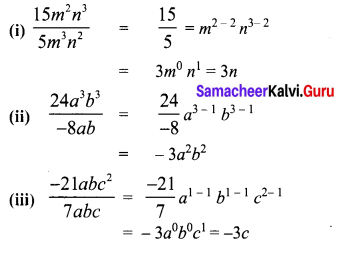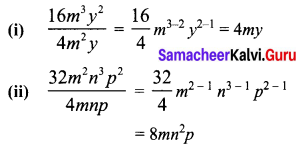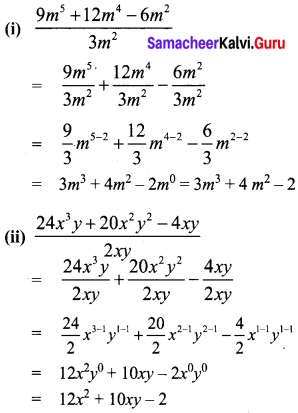Students can Download Maths Chapter 3 Algebra Additional Questions and Answers, Notes Pdf, Samacheer Kalvi 8th Maths Book Solutions Guide Pdf helps you to revise the complete Tamilnadu State Board New Syllabus and score more marks in your examinations.## Tamilnadu Samacheer Kalvi 8th Maths Solutions Term 1 Chapter 3 Algebra Additional Questions

Exercise 3.1

Question 1.
Find the product of the following.
(i) (x, y)
(ii) (10x, 5y)
(iii) (2x2, 5y2)
Solution:
(i) x × y = xy
(ii) 10x × 5y = (10 × 5) x × xy
= 50 xy
(iii) 2x2 x 5y2 = (2 x 5) x (x2 + y2)
= 10x2y2

Question 1.
Find the product of the following.
(i) 3ab2 c3 by 5a3b2c
(ii) 4x2yz by $$\frac{3}{2}$$ x2yz2
Solution:
(i) (3ab3c3) × (5a3b2c)
= (3 × 5)(a × a3 × b2 × b2 × c2 × c)
= 15a1+3.b2+2.c3+1 = 15a4b4c4

(ii) 4x2yz by $$\frac{3}{2}$$ x2yz2
= (4 × $$\frac{3}{2}$$) × (x2 × x2 × y × y × z × z2)
= -6x2+2 y1+1 x1+2 = -6x4y2z3

Question 1.
Simplify (3x – 2) (x – 1) (3x + 5).
Solution:
(3x – 2) (x – 1) (3x + 5)
= {(3x – 2) (x – 1)} × (3x + 5) [∴Multiplication in associative]
= {3x (x – 1) – 2 x – 1)} × (3x + 5)
= (3x2 – 3x – 2x + 2) × (3x + 5)
= (3x2 – 5x + 2) (3x + 5)
= 3x2 × (3x + 5) – 5x (3x + 5) + 2 (3x + 5)
= (9x3 + 15x2) + (-15x2 – 25x) + (6x + 10)
= 9x3 + 15x2 – 15x2 – 25x + 6x + 10
= 9x3 – 19x + 10Question 2.
Simplify (5 – x) (3 – 2x) (4 – 3x).
Solution:
(5 – x) (3 – 2x) (4 – 3x)
= {(5 – x)(3 – 2x)} × (4 – 3x) [∴ Multiplication in association]
= {5 (3 – 2x) -x (3 – 2x)} × (4 – 3x)
= (15 – 10x – 3x + 2x2) × (4 – 3x)
= (2x2 – 13x + 15) (4 – 3x)
= 2x2 × (4 – 3x) – 13x (4 – 3x) + 15 (4 – 3x)
= 8x3 – 63 – 52x + 39x2 + 60 – 45x
= -6x3 + 47x2 – 97x + 60

Exercise 3.2

Question 1.
Divide.
(i) 12x3y3 by 3x2y
(ii) -15a2 bc3 by 3ab
(iii) 25x3y2 by – 15x2y
Solution:Question 1.
Divide
(i) 15m2n3 by 5m2n2
(ii) 24a3b3 by -8ab
(iii) -21 abc2 by 7 abc
Solution:Question 2.
Divide
(i) 16m3y2 by 4m2y
(ii) 32m2 n3p2 by 4mnp
Solution:Question 1.
Divide.
(i) 9m5 + 12m4 – 6m2 by 3m2
(ii) 24x3y + 20x2y2 – 4xy by 2xy
Solution:Exercise 3.3

Question 1.
Evaluate:
(i) (2x + 3y)2
(ii) (2x – 3y)2
Solution:
(i) (2x + 3y)2
= (2x)2 + 2 × (2x) × (3y) + (3y)2
[using (a + b)2 = a2 + 2ab + b2]
= 4x2 + 12xy + 9y2

(ii) (2x – 3y)2
= (2x)2 – 2(2x) (3y) + (3y)2
[∵ using (a – b)2 = a2 – 2ab + b2]
= 4x2 – 12xy + 9y2

Question 1.
Evaluate the following
(i) (2x – 3) (2x + 5)
(ii) (y – 7) (y + 3)
(iii) 107 × 103
Solution:
(i) (2x – 3) (2x + 5)
= (2x)2 + (-3 + 5) (2x) + (-3) (5)
[∵ (x + a) (x + b) = x2 + (a + b)x + ab]
= 22x2 + 2 × 2x + (-15)
= 4x2 + 4x – 15

(ii) (y – 7) (y + 3)
= y2 + (-7 + 3)y + (-7) (3)
[∵ (x + a)(x + b) = x2 + (a + b)x + ab]
= y2 – 4y + (-21) = y2 – 4y – 21

(iii) 107 × 103
= (100 + 7) × (100+ 3)
= 1002 + (7 + 3) × 100 +(7 × 3)
= 10000 + 10 × 100 + 21 = 10000 + 1000 + 21 = 11021Question 1.
If x + y = 12 and xy = 14 find x2 + y2.
Solution:
(x + y)2 = x2 + y2 + 2xy
122 = x2 + y2 + 2 × 14
144 = x2 + y2 + 28
x2 + y2 = 144 – 28
x2 + y2 = 116

Question 2.
If 3x + 2y = 12 and xy = 6 find the value of 9x2 + 4y2.
Solution:
(3x + 2y)2 = (3x)2 + (2y)2 + 2 (3x) (2y)
= 9x2 + 4y2 + 12xy
122 = 9x2 + 4y2 + 12 × 6
144 = 9x2 + 4y2 + 72
144 – 72 = 9x2 + 4y2
∴ 9x2 + 4y2 = 72

Exercise 3.4

Question 1.
Factorize:
(i) 7(2x + 5) + 3 (2x + 5)
(ii) 12x3y4 + 16x2y5 – 4x5y2
Solution:
(i) 7(2x + 5) + 3 (2x + 5)
= (2x + 5) (7 + 3)
(ii) 12x3y4 + 16x2y5 – 4x5y2
= 4x2y2 (3xy2 + 4y3 – x3)

1. Factorize
(i) 81a2 – 121b2
(ii) x2 + 8x + 16
Solution:
(i) 81a2 – 121b2
= (9a)2 – (11b)2
[∵ using a2 – b2 = (a + b)2]
= (9a + 11b) (9a – 11b)

(ii) x2 + 8x + 16 = x2 + 2 × x × 4 + 42
[∵ using a2 + 2ab + b2 = (a + b)2]
= (x + 4)2 = (x + 4)(x + 4)

Question 1.
Factorize
(i) x2 + 2xy + y2 – a2 + 2ab – b2
(ii) 9 – a6 + 2a3 – b6
Solution:
(i) x2 + 2xy + y2 – a2 + 2ab – b2.
= (x2 + 2xy + y2) – (a2 – 2ab + b2)
= (x + y)2 – (a – b)2
= {(x + y) + (a – b)} {(x + y) – (a – b)}
= (x + y + a – b) (x + y – a + b)

(ii) a – a6 + 2a3b3 – b6
= 9 – (a6 – 2a3b3 + b6)
= 32 -{(a3)2 – 2 × a3 × b3 + (b3)2}
= 32 – (a3 – 63)2
= {3 + (a3 – b3)} {3 – (a3 – b3)}
= (3 + a3 – b3) (3 – a3 + b3)
= (a3 – b3 + 1){-a3 + b3 + 3)

Question 2.
Factorize
(i) 100 (x + y )2 – 81 (a + b)2
(ii)(x + 1)2 – (x – 2)2
Solution:
(i) 100 (x + y)2 – 81 (a + b)2
= {10 (x + y)}2 – {(a (a + b)}2
= {10 (x + y) + 9 (a + b)}
{10 (x + y) – 9(a + b)}
= (10x + 10y + 9a – 9b)}
(10x + 10y – 9a – 9b)

(ii) (x – 1)2 – (x – 2)2
= {(x – 1 +(x – 2)}
{(x – 1) – (x – 2)}
= (2x – 3) – (x – 1 – x + 2)
= (2x – 3) × 1 = 2x – 3